## Voltage Profile of Transmission Line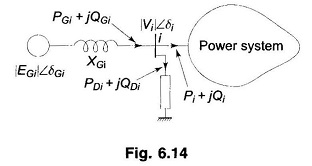Voltage Profile of Transmission Line: Control of Voltage Profile of Transmission Line at the receiving bus in the fundamental two-bus system was discussed already. Though the same general conclusions hold for an interconnected system, it is important to discuss this problem in greater detail. At a bus with generation, voltage can be conveniently controlled by …

## Comparison of Gauss Seidel and Newton Raphson Load Flow Methods

Comparison of Gauss Seidel and Newton Raphson Load Flow Methods: Comparison of Gauss Seidel and Newton Raphson Load Flow Methods, when both use YBUS as the network model. It is experienced that the Gauss Seidel method works well when programmed using rectangular coordinates, whereas Newton Raphson requires more memory when rectangular coordinates are used. Hence, polar …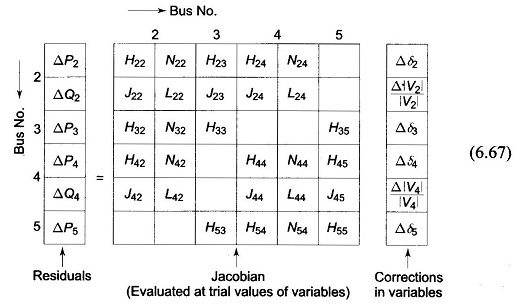Decoupled Load Flow Methods (DLF): An important characteristic of any practical electric power transmission system operating in steady state is the strong interdependence between real powers and bus voltages angles and between reactive powers and voltage magnitudes. This interesting property of weak coupling between P-δ and Q-V variables gave the necessary motivation in developing the …

## Newton Raphson Method for Load Flow Analysis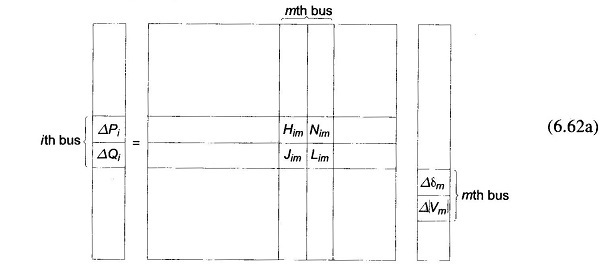Newton Raphson Method for Load Flow Analysis: The Newton Raphson Method for Load Flow Analysis is a powerful method of solving non-linear algebraic equations. It works faster and is sure to converge in most cases as compared to the GS method. It is indeed the practical method of load flow solution of large power networks. …

## Load Flow Analysis in Power System

Load Flow Analysis in Power System: Load Flow Analysis in Power System describes, the complex power injected by the source into the ith bus of a power system is where Vi is the voltage at the ith bus with respect to ground and Ji is the source current injected into the bus. The Load Flow …

## Formation of YBUS By Singular Transformation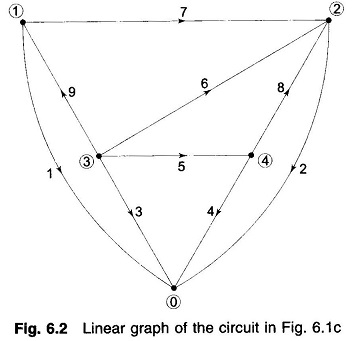Formation of YBUS By Singular Transformation: Formation of YBUS By Singular Transformation – The matrix pair YBUS and ZBUS form the network models for load flow studies. The YBUS can be alternatively assembled by use of singular transformations given by a graph theoretical approach. This alternative approach is of great theoretical and practical significance and …

## One Line Diagram of the 4 Bus System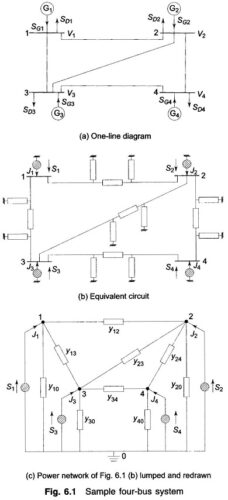One Line Diagram of the 4 Bus System: A power system comprises several buses which are interconnected by means of transmission lines. Power is injected into a bus from generators, while the loads are tapped from it. Of course, there may be buses with only generators and no-loads, and there may be others with only …

Scroll to Top